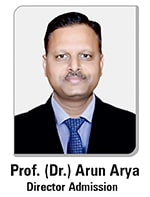## Prof. (Dr.) Arun Arya

•9314881683 | 9829017324
•0141-6604555 (30 Lines)
Toll Free: 1800 266 2000
•info@aryacollege.in## Prof. (Dr.) Arun Arya

•9314881683 | 9829017324
•0141-6604555 (30 Lines)
Toll Free: 1800 266 2000
•info@aryacollege.in

# What type of Mathematics is required to become an engineer?

## Mathematics subjects to include in the engineering curriculum

For an individual, acquiring an engineering degree requires to complete a multitude of math courses successfully. In the earlier days, engineers used slide rules and pencils to work out math problems. In today’s world, engineers of Top Engineering Colleges still need a solid foundation in math and a good understanding of mathematical principles. Even after planning the study pattern and strategies, most of the colleges still require a particular set of mathematics courses.

### Algebra

Every university or college has different requirements. But a thorough understanding of algebra is crucial to success in advanced math courses. An individual may need to take one or two semesters of algebra and trigonometry in their college. At some schools, if an individual had high school algebra and trigonometry, they are deemed proficient enough to begin their math with calculus. Other schools look at their placement test scores or math scores on their SAT or ACT.

### Calculus

Most engineering degree plans require three semesters of calculus. In conjunction with advanced calculus, an individual of the top B Tech Colleges need to take analytic geometry. Analytic geometry uses the principles of calculus and trigonometry to determine limits, vectors, integrals, mean values and derivatives. One of the more advanced math functions engineers must understand is differential equations.

### Trigonometry

Trigonometry is one of the more usual maths for engineers. By applying the principle of trigonometry, engineers can calculate such data as the height of an existing structure, the measurement of an angle, or the distance between two points. In other words, trigonometry is similar to plane geometry. For instance, it relies heavily on formulas and logarithms, rather than solving complex equations.

### Probability and Statistics

Some of the Engineering Colleges in Jaipur separate probability and statistics into two separate courses, but other schools combine them. Probability teaches an individual how to calculate the odds of an event occurring, with random variables and the various theories on limits. Statistics cover sampling techniques, estimation, the margin of error and other skills needed to analyze data.

#### Conclusion

Depending on the engineering specialty, engineers may take additional mathematics courses. For instance, an individual might have to devote an entire class to vectors. Arya College offers engineering math courses that combine elements of multiple classes. Discrete mathematics uses the theory of sets, along with equations and graphs to describe algorithms and data.

## Latest Posts

• ### MBA: Course, Syllabus, Subjects, Fees, Salary & Scope

June 05, 2023
• ### Which Specialization is best in MBA after BBA?

June 01, 2023

May 11, 2023
• ### Future of Data Science: Full Guide

April 28, 2023
• ### 10 Leadership Skills for MBA Students

April 25, 2023